[BACK]
 Computers, Materials & ContinuaDOI:10.32604/cmc.2022.023682Article

SSABA: Search Step Adjustment Based Algorithm

1Department of Mathematics and Computer Sciences, Sirjan University of Technology, Sirjan, Iran
2Department of Civil Engineering, Islamic Azad University, Estahban Branch, Estahban, Iran
3Department of Mathematics, Faculty of Science, University of Hradec Králové, Hradec Králové, 500 03, Czech Republic
4Department of Electrical and Electronics Engineering, Shiraz University of Technology, Shiraz, Iran
5Department of Applied Cybernetics, Faculty of Science, University of Hradec Králové, Hradec Králové, 500 03, Czech Republic
6Department of Computer Science, Government Bikram College of Commerce, Patiala, Punjab, India
*Corresponding Author: Pavel Trojovský. Email: pavel.trojovsky@uhk.cz
Received: 17 September 2021; Accepted: 20 October 2021

Abstract: Finding the suitable solution to optimization problems is a fundamental challenge in various sciences. Optimization algorithms are one of the effective stochastic methods in solving optimization problems. In this paper, a new stochastic optimization algorithm called Search Step Adjustment Based Algorithm (SSABA) is presented to provide quasi-optimal solutions to various optimization problems. In the initial iterations of the algorithm, the step index is set to the highest value for a comprehensive search of the search space. Then, with increasing repetitions in order to focus the search of the algorithm in achieving the optimal solution closer to the global optimal, the step index is reduced to reach the minimum value at the end of the algorithm implementation. SSABA is mathematically modeled and its performance in optimization is evaluated on twenty-three different standard objective functions of unimodal and multimodal types. The results of optimization of unimodal functions show that the proposed algorithm SSABA has high exploitation power and the results of optimization of multimodal functions show the appropriate exploration power of the proposed algorithm. In addition, the performance of the proposed SSABA is compared with the performance of eight well-known algorithms, including Particle Swarm Optimization (PSO), Genetic Algorithm (GA), Teaching-Learning Based Optimization (TLBO), Gravitational Search Algorithm (GSA), Grey Wolf Optimization (GWO), Whale Optimization Algorithm (WOA), Marine Predators Algorithm (MPA), and Tunicate Swarm Algorithm (TSA). The simulation results show that the proposed SSABA is better and more competitive than the eight compared algorithms with better performance.

Keywords: Optimization; population-based; optimization problem; search step; optimization algorithm; minimization; maximization

1  Introduction

Optimization is the process of presenting the best solution to a problem out of all the possible solutions to that problem given the limitations and needs of the problem . From a mathematical point of view, an optimization problem can be modeled using the three main parts of decision variables, constraints, and objective functions . Methods for solving optimization problems fall into two groups: deterministic methods and stochastic methods .

Deterministic methods using derivative and Gradient information of objective functions as well as mathematical operators are able to provide optimal solutions to optimization problems. The main advantage of deterministic methods is that they guarantee the proposed solution as the optimal solution. The inability of these methods to solve non-derivative problems, high computational volume in complex problems, time consuming in solving problems with high number of variables, and convergence dependence on initial values in methods that depend on the initial conditions are the most important disadvantages of deterministic methods .

On the other hand, optimization algorithms as stochastic methods of solving optimization problems, are able without the need for derivative and gradients information with using random operators and random scanning of the problem search space, in a repetition-based process provide appropriate and acceptable solutions for optimization problems . Optimization algorithms are inspired by the concepts of biology, animal behavior, physics, games, and other processes that can be modeled as optimizers . For example, in design of Ant Colony Optimization (ACO) is inspired by the ant colony behavior in locating food and how to find the shortest path between food sources and nests . Gravitational Search Algorithm (GSA) is inspired by the physical fundamentals of Newton's laws of motion and the gravitational force . Darts Game Optimizer (DGO) is inspired by simulating the rules of the darts game and the behavior of the players in this game .

In optimization studies, global optimal solution is the main solution to the optimization problem. Deterministic methods, in optimizing problems that can be implemented, are able to provide this global optimal solution. Stochastic-based optimization algorithms do not necessarily provide the global optimal solution. However, the solutions obtained with optimization algorithms should be as close as possible to the global optimal. For this reason, the solutions obtained using optimization algorithms are called quasi-optimal . The concept of quasi-optimal solutions and the desire to improve them to better quasi-optimal and closer to global optimal solution has led to the design of numerous optimization algorithms by researchers. In this regard, these optimization algorithms are applied in various fields in the literature such as energy , protection , electrical engineering , and energy carriers [22,23] to achieve the optimal solution.

The contribution of this paper is the introduction and design of a new optimization algorithm called Search Step Adjustment Based Algorithm (SSABA) in order to provide quasi-optimal solutions to various optimization problems. Setting the search step in the problem-solving space during the iteration of the algorithm is the main idea in designing the proposed SSABA. In fact, by adjusting the search step, a member of the population, in the initial iterations of the algorithm, scans different spaces of the search space, and as the number of iterations of the algorithm increases, the search step becomes smaller in order to increase the exploitation power. The theory of the proposed SSABA is described and then mathematically modeled. The performance of SSABA is implemented on twenty-three standard objective functions of different types of unimodal and multimodal and is also compared with eight well-known algorithms.

The rest of this paper is organized in such a way that a study on optimization algorithms is presented in Section 2. The proposed SSABA is introduced and modeled in Section 3. Simulation studies are presented in Section 4. Conclusions and several suggestions for future studies are provided in Section 5.

2  Background

Optimization algorithms use a relatively similar mechanism to discover the optimal solution. Initially, a number of feasible solutions are generated randomly considering the limitations of the problem. Then, based on the different steps of the algorithm, in an iteration-based process, these initial solutions are improved. The process of updating and improving the proposed solutions continues until the algorithm stop criterion is reached. The distinguishing point of optimization algorithms is the main design idea and mechanism used in updating the proposed solutions. Accordingly, optimization algorithms can be divided based on the main design idea into four groups: swarm-based, physics-based, evolutionary-based, and game-based .

2.1 Swarm-Based Optimization Algorithms

Swarm-based optimization algorithms are designed based on simulation of the swarming behaviors of living organisms such as animals, plants, and insects. Particle Swarm Optimization (PSO) can be mentioned as one of the oldest and most widely used algorithms in this group. PSO is inspired by the social behavior of animals such as fish and birds that live together in small and large groups. In PSO, population members communicate with each other and solve problems using the experience of the best member of the population as well as the best personal experience . Grey Wolf Optimization (GWO) is one of the swarm-based algorithms to solve optimization problems that is inspired by the group life of wolves and how they hunt in nature. In GWO, the hierarchical behavior of leadership in the group of gray wolves is modeled using four different types of wolves. The status of each member of the population is updated as a solution to the problem based on the modeling of the hunting process in three phases tracking the prey, encircling the prey, and attacking the prey . Whale Optimization Algorithm (WOA) is a swarm-based optimization algorithm which is designed by inspiring the nature and social behavior of humpback whales in a bubble network hunting strategy to achieve a quasi-optimal solution . Some other algorithms that belong to this group are: Teaching-Learning Based Optimization (TLBO) , Marine Predators Algorithm (MPA) , Tunicate Swarm Algorithm (TSA) , Emperor Penguin Optimizer (EPO) , Following Optimization Algorithm (FOA) , Group Mean-Based Optimizer (GMBO) , Donkey Theorem Optimization (DTO) , Multi Leader Optimizer (MLO) , Two Stage Optimization (TSO) , Mixed Leader Based Optimizer (MLBO) , Good Bad Ugly Optimizer (GBUO) , Mixed Best Members Based Optimizer (MBMBO) , Teamwork Optimization Algorithm (TOA) , and Good and Bad Groups-Based Optimizer (GBGBO) .

2.2 Physics-Based Optimization Algorithms

Physics-based optimization algorithms are developed based on the modeling of various physical laws and phenomena. Simulated Annealing (SA) is one of the well-known methods of this group of optimization algorithms, which is designed based on simulation of annealing process in metallurgy . Hook's law simulation in a system consisting of weights and springs connected to each other is used in the design of Spring Search Algorithm (SSA) . Momentum Search Algorithm (MSA) is introduced based on the modeling of momentum law and Newtonian laws of motion . Some other algorithms that belong to this group are: Black Hole (BH) , Ray Optimization (RO)  algorithm, Magnetic Optimization Algorithm (MOA) , Galaxy-based Search Algorithm (GbSA) , Charged System Search (CSS) , Artificial Chemical Reaction Optimization Algorithm (ACROA) , Binary Spring Search Algorithm (BSSA) , Curved Space Optimization (CSO) , and Small World Optimization Algorithm (SWOA) .

2.3 Evolutionary-Based Optimization Algorithms

Evolutionary-based optimization algorithms are developed based on the modeling of genetics science, biology science and evolutionary operators. Genetic Algorithm (GA) is definitely the most well-known method of intelligent optimization and evolutionary algorithm that has many applications in various scientific and engineering disciplines. GA is introduced based on mathematical modeling of the reproductive process and Darwin's theory of evolution. The process of updating population members of chromosomes, each of which is a solution to the problem, is simulated using three operators: selection, crossover, and mutation . Artificial Immune System (AIS) algorithms are among the algorithms inspired by the mechanism of the human body that fall into the category of evolutionary systems. The human immune system is one of the most accurate and astonishing living systems which modeling the mechanism of this system against viruses and microbes has been used in the design of AIS . Some other algorithms that belong to this group are: Biogeography-based Optimizer (BBO) , Differential Evolution (DE) , Genetic Programming (GP) , and Evolution Strategy (ES) .

2.4 Game-Based Optimization Algorithms

Game-based optimization algorithms are developed based on the modeling of rules of different games and the behavior of players in those games. HOGO is designed based on simulating the process of searching players to find a hidden object. In HOGO, players are the solution to a problem that moves through the search space to try to find the hidden object, which is actually the optimal solution to the problem . FGBO is developed based on mathematical modeling of the behavior of players and clubs in the game of football . Orientation Search algorithm (OSA) is introduced based on the simulation of players’ movement in the direction specified by the referee . Some other algorithms that belong to this group are: Dice Game Optimizer (DGO) , Ring Toss Game Based Optimizer (RTGBO) , and Binary Orientation Search algorithm (BOSA) .

3  Search Step Adjustment Based Algorithm

In this section, the theory of the proposed Search Step Adjustment Based Algorithm (SSABA) is described and its mathematical modeling is presented for use in solving optimization problems.

The proposed SSABA is a stochastic and evolutionary-based algorithm for solving optimization problems. In population-based algorithms, each member of the population represents a solution to the optimization problem that contains information and values of decision variables. Initially, a number of possible solutions to the problem are generated randomly. Then, in an iterative process, the initial solutions are improved to converge towards the optimal global solution to the problem. In each iteration, population members suggest new values for the problem variables by randomly moving through the search space. The main idea of the SSABA in updating the position of population members in the search space is to set the search step during successive iterations of the algorithm.

In the proposed SSABA, each population member can be modeled using a vector and the whole population of the algorithm using a matrix based on Eq. (1).

X=[X1XiXN]N×m=[x1,1x1,dx1,m...xi,1xi,dxi,m...xN,1xN,dxN,m]N×m,(1)

where X is the population matrix of SSABA, Xi is the ith population member, N is the number of population members, m is the number of problem variables, xi,d is the dth dimension of status of ith population member in search space.

The objective function of the optimization problem can be evaluated based on the values for the problem variables which are proposed by each population member. The calculated values for the objective function can be modeled using a vector based on Eq. (2).

F=[F1FiFN]N×1=[F(X1)F(Xi)F(XN)]N×1,(2)

where F is the objective function vector and Fi is the objective function value which is evaluated based on proposed problem variables by the ith population member.

Based on the comparison and analysis of the values obtained for the objective function, the member that provides the best value for the objective function is determined as the best member of the population. The best member of the population as a leader is able to lead the members of the population in the search space towards the suitable solution. Therefore, in the proposed SSABA, the population members move towards the best population member. In this update process, the search step is set during the iterations of the algorithm. The mechanism of adjusting the search step is such that in the initial iterations that the algorithm must scan different areas of the search space well, it is set to its maximum value. Then, as the replication of the algorithm increases, and the population members approach the optimal global solution, the search step value decreases so that the population members converge with smaller and more accurate steps towards the global optimal solution. This search step adjustment mechanism is simulated using Eq. (3).

St=2tT,(3)

where St is the search step in the tth iteration of algorithm, t is the counter of iterations, and T is the maximum number of iterations.

After adjusting the search step in each iteration, the members of the population are updated in the search space according to the search step, and based on the guidance of the best member of the population. The update process in the proposed SSABA is mathematically modeled using Eqs. (4)(6). The new suggested position for each population member in the search space is determined based on the main idea of the proposed SSABA, which is to move towards the best member of the population by adjusting the search step, using Eqs. (4) and (5). This new position is acceptable to a population member if the value of the objective function improves in that new position, otherwise the member remains in its previous position. This process is simulated using Eq. (6).

Xinew:xi,dnew=xi,d+Str(xbestIxi,d),(4)

I=round(1+r),(5)

Xi={Xinew,FinewFi;Xi,else,(6)

where Xinew is the new suggested status for the ith population members, xi,dnew is its dth dimension, Finew is its objective function value, r is a random number in the interval [0, 1], xbest is the best population member, and I is a random number which is equal to 1 or 2.

After updating all members of the population, the algorithm enters the next iteration. Based on the new values, the best member of the population is updated. Then the search step for the new iteration is calculated and the population members are updated according to Eqs. (4)(6). This update process continues until the end of the algorithm iterations. At the end and after the complete implementation of the algorithm, the best quasi-optimal solution to which the SSABA has converged is presented as the solution of the problem. the pseudocode of the SSABA is presented in Algorithm 1. Also, the various stages of the proposed SSABA are shown as flowcharts in Fig. 1.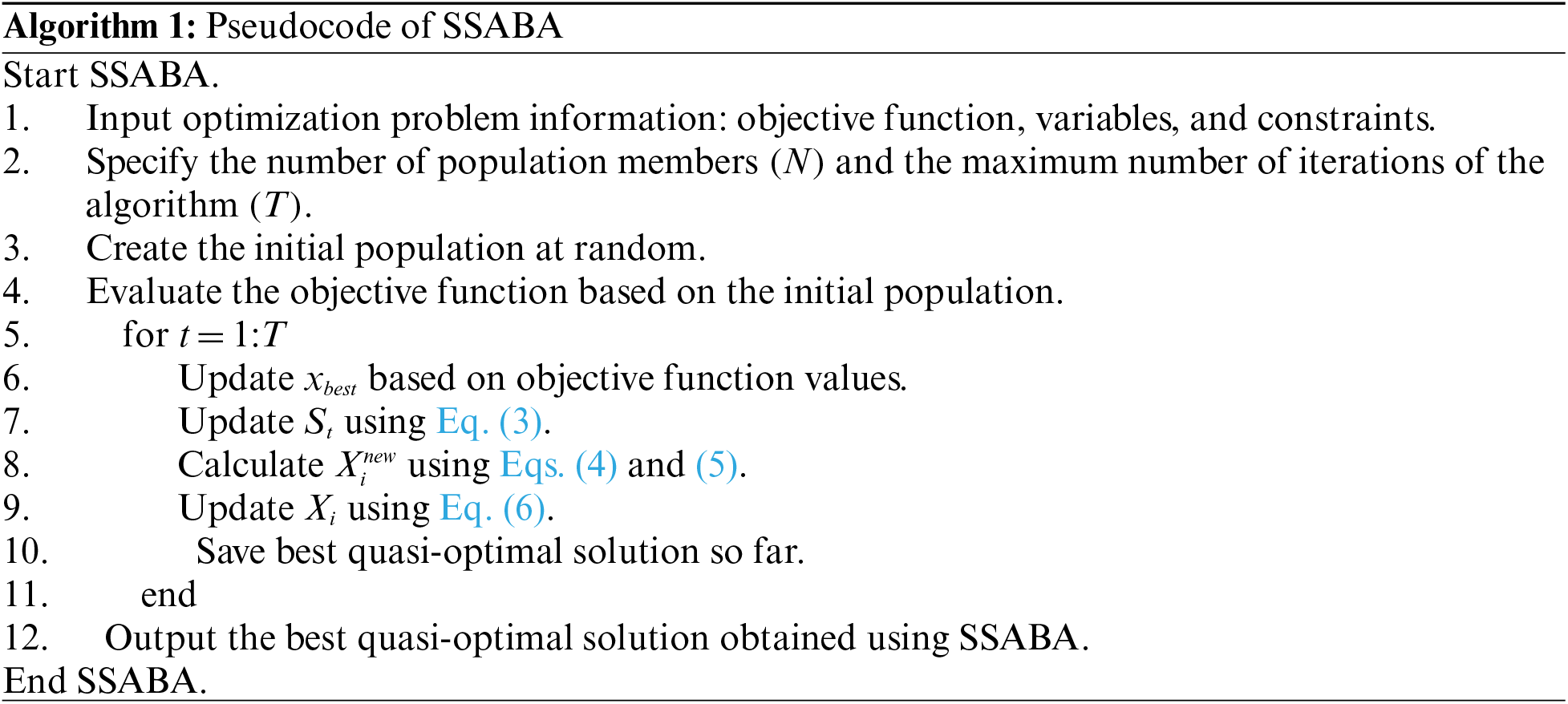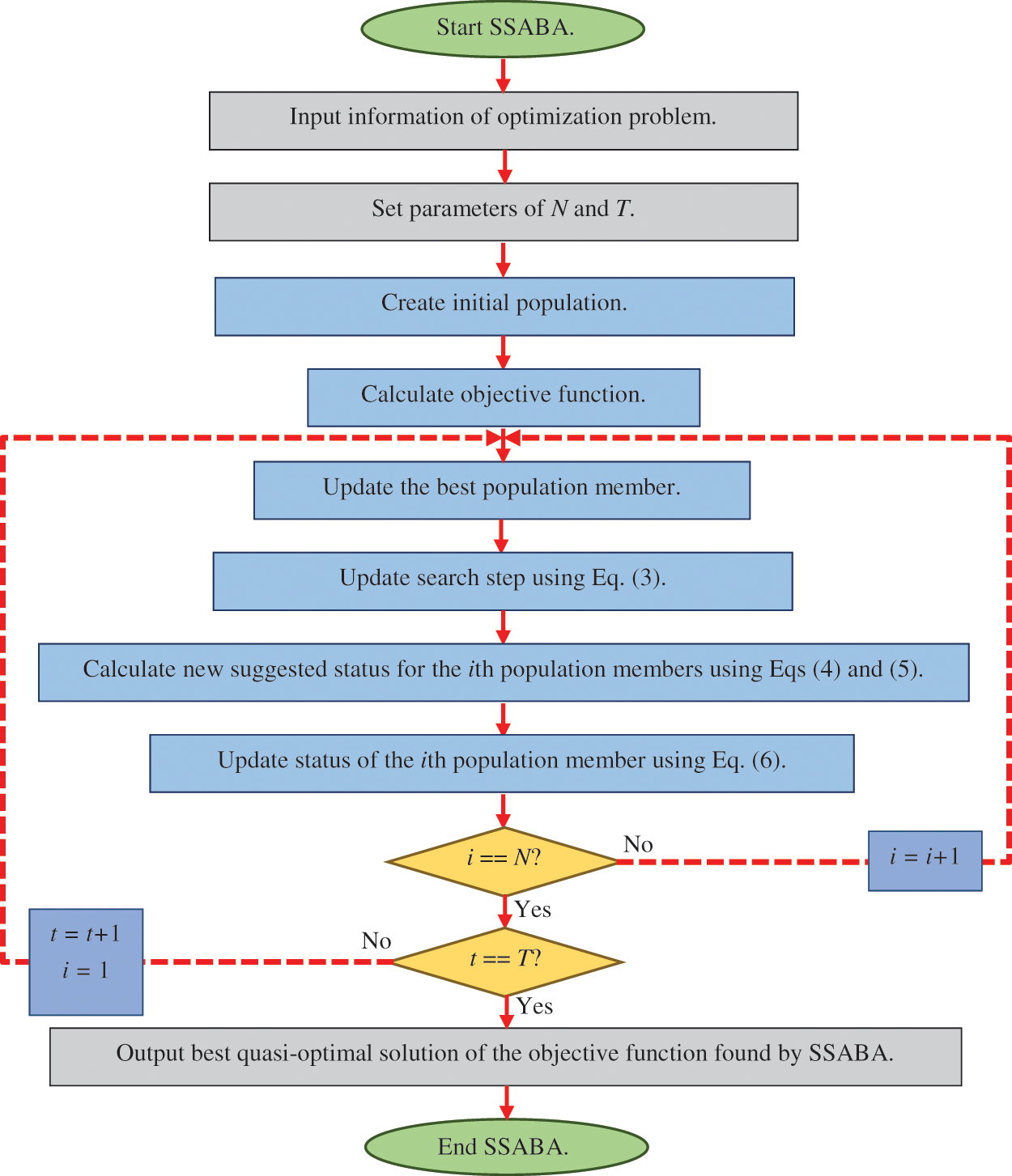Figure 1: Flowchart of SSABA

The time complexity of the proposed SSABA can be represented as:

1.    The initialization of population process needs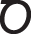(N × m) time, where N is the number of population members and m is the number of dimensions of a given optimization problem.

2.    The population member fitness needs(T × N × m) time, where T is the maximum number of iterations.

3.    Steps 1 and 2 are repeated until the satisfactory results is found which needs(S) time.

Thus, the overall time complexity of SSABA is(T ×N × m ×S).

4  Simulation Studies

Simulation studies and performance analysis of the proposed SSABA in optimization are presented in this section. SSABA is implemented on a standard set consisting of twenty-three objective functions of different types of unimodal, high-dimensional multimodal, and fixed-dimensional multimodal. Complete information on these objective functions is provided in Appendix A and in Tabs. A1A3. In order to analyze the ability of SSABA in optimization, the optimization results obtained from it are compared with eight well-known algorithms including Particle Swarm Optimization (PSO) , Genetic Algorithm (GA) , Teaching-Learning Based Optimization (TLBO) , Gravitational Search Algorithm (GSA) , Grey Wolf Optimization (GWO) , Whale Optimization Algorithm (WOA) , Marine Predators Algorithm (MPA) , Tunicate Swarm Algorithm (TSA) . The simulation results of the optimization algorithms in solving the objective functions are reported using two indicators of the average of the best obtained solutions (ave) and the standard deviation of the best obtained solutions (std).

4.1 Evaluation of Objective Functions

4.1.1 Unimodal Functions

The first type of functions considered to evaluate the performance of optimization algorithms are seven unimodal functions, including F1 to F7. The optimization results of these objective functions using the SSABA and eight compared algorithms are presented in Tab. 1. Based on the analysis of the optimization results, it is clear that the SSABA is able to provide the optimal global solution for F1 and F6 functions. SSABA is also the best optimizer for optimizing F2, F3, F4, F5, and F7 functions. The simulation results of F1 to F7 functions show that the proposed SSABA is far superior to the eight compared algorithms.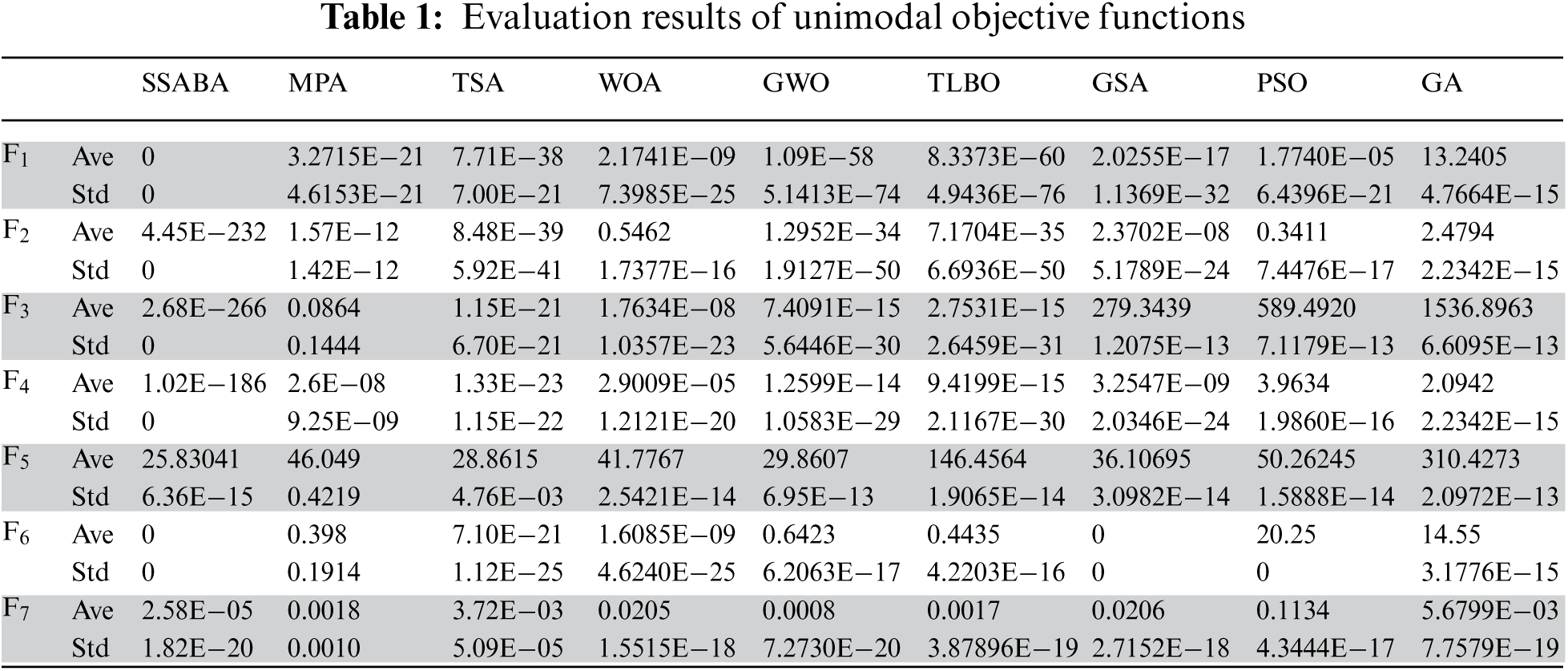4.1.2 High-Dimensional Multimodal Functions

The second type of functions considered for analyzing the performance of optimization algorithms are six high-dimensional multimodal functions including F8 to F13. The results of the implementation of SSABA and eight comparative algorithms on high-dimensional multimodal functions are presented in Tab. 2. What can be seen from the analysis of the results in this table is that the proposed SSABA provides the optimal global solution for the F9 and F11 functions. SSABA is the best optimizer for F8, F10, and F12 functions. Analysis of the performance of optimization algorithms in solving F8 to F13 functions shows that SSABA has a high capability in solving such optimization problems and is superior to the compared algorithms.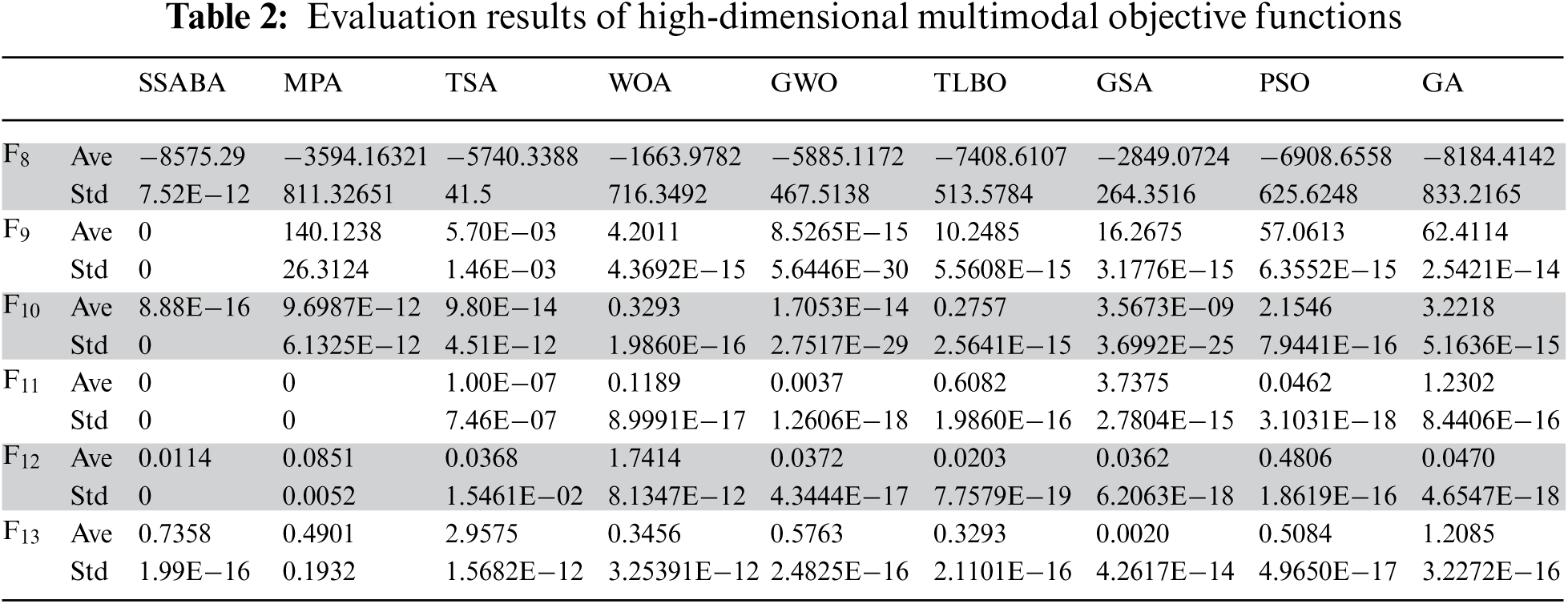4.1.3 Fixed-Dimensional Multimodal Functions

The third type of selective functions to analyze the capabilities of optimization algorithms are ten fixed-dimensional multimodal functions including F14 to F23. The results of optimization of F14 to F23 objective functions using SSABA and eight compared algorithms are presented in Tab. 3. The optimization results show that SSABA has been able to provide the global optimal solution for the F17 function. SSABA is also the best optimizer for F15, F16, F19, and F20 functions. The proposed SSABA with less standard deviation, in F14, F18, F21, F22, and F23 functions has a better performance than similar algorithms. Analysis of the simulation results shows that SSABA has a high ability to solve fixed-dimensional multimodal optimization problems and is much more competitive than the eight compared algorithms.

4.2 Discussions

Exploitation and exploration are two factors influencing the performance of optimization algorithms in achieving appropriate solutions to optimization problems.

The concept of exploitation in the study of optimization algorithms shows the ability of the algorithm to search locally and get closer to the global optimal solution. In fact, an algorithm that has the suitable conditions in the power of exploitation is able to converge to a solution closer to the global optimal. The exploitation ability is especially important in solving optimization problems that have only one main solution without having local optimal areas. Unimodal functions with such features are very suitable for analyzing the ability of exploitation to achieve suitable quasi-optimal solutions. The results of optimization of the F1 to F7 Unimodal objective functions presented in Tab. 1 indicate that the proposed SSABA with high exploitation capability has been converged to suitable solutions that are very close to the global optimal. The performance analysis of the compared algorithms shows that SSABA is much more competitive than them in terms of exploitation capability.

The concept of exploration in the study of optimization algorithms means the ability of the algorithm to globally search and accurately scan the problem search space. In fact, an algorithm must be able to scan different areas of the search space in order to explore the optimal solution. The exploration ability is especially important in implementing algorithms on problems that have optimal local solutions. Multimodal objective functions of both types of high-dimensional, including F8 to F13 and fixed-dimensional including F14 to F23, have several local solutions in addition to the main solution. Therefore, these types of functions are very suitable for evaluating the exploration power of optimization algorithms. What can be deduced from the analysis of the optimization results of this type of function presented in Tabs. 2 and 3 is that the proposed SSABA with high ability in exploration power has been able to scan different areas of the search space and, as a result, cross local optimal solutions. Analysis of the simulation results shows that SSABA has more appropriate exploration power than the compared algorithms and is able to scan the problem search space more effectively.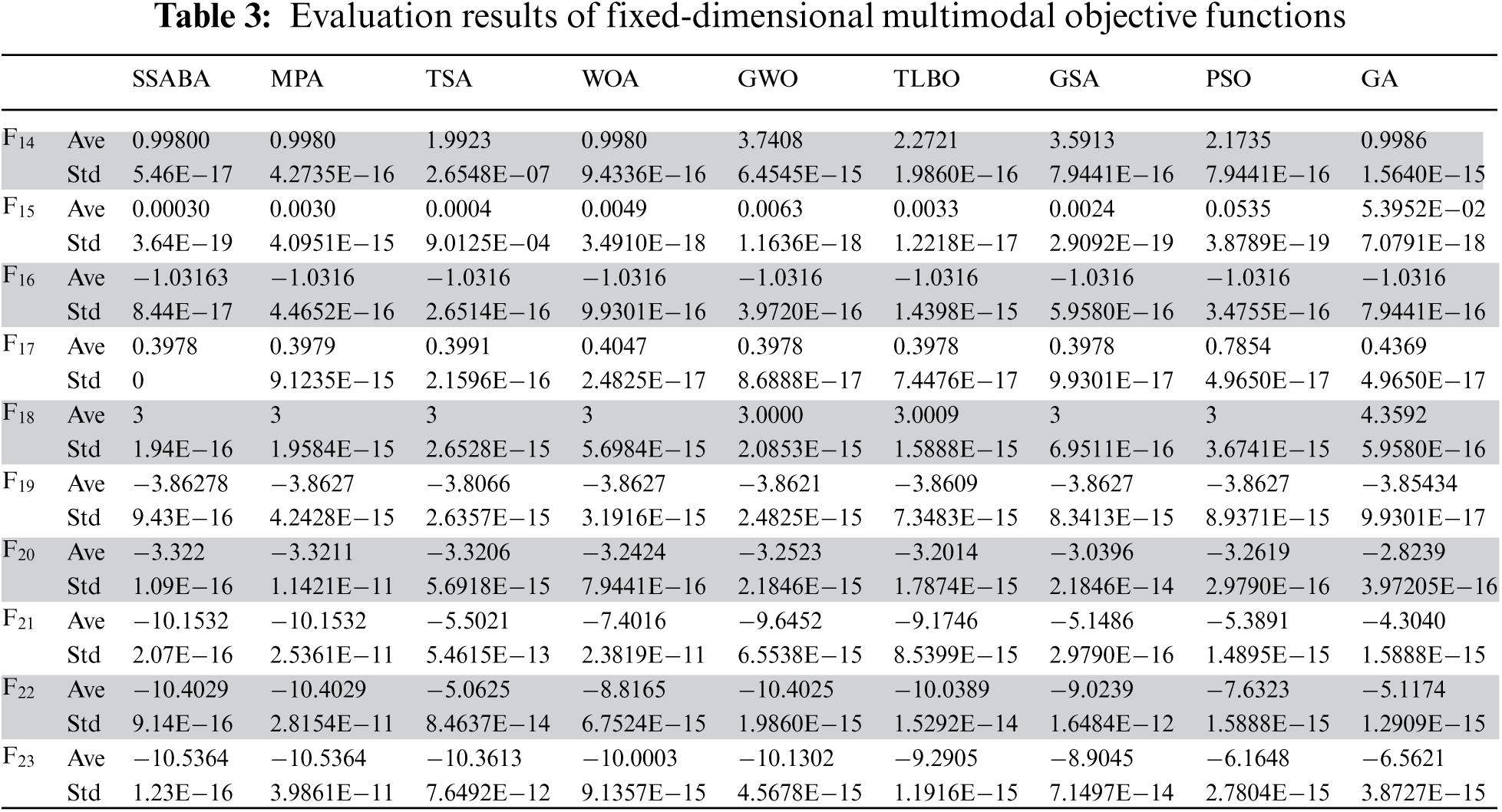4.3 Statistical Analysis

In this subsection, a statistical analysis on the performance of optimization algorithms in solving optimization problems is presented. Analysis of optimization algorithms based on the two criteria of the average of the best solutions as well as the standard deviation of the best solutions provides valuable information about their ability. However, even after twenty independent runs, it is possible for an algorithm to be superior to compared algorithms at random. Therefore, in this study, the Wilcoxon sum-rank test  is used to analyze the superiority of the proposed SSABA from a statistically significant point of view. Wilcoxon sum-rank test is a non-parametric test that is used to compare and assess the similarity of two independent samples.

The results of statistical analysis of SSABA against the compared algorithms using Wilcoxon sum-rank test are presented in Tab. 4. In the Wilcoxon sum-rank test, an index called p-value is used to detect statistical differences between the two data sets. Based on the analysis of the results presented in Tab. 4, it is concluded that the superiority of SSABA is statistically significant against the compared algorithm in cases where the p-value is less than 0.05.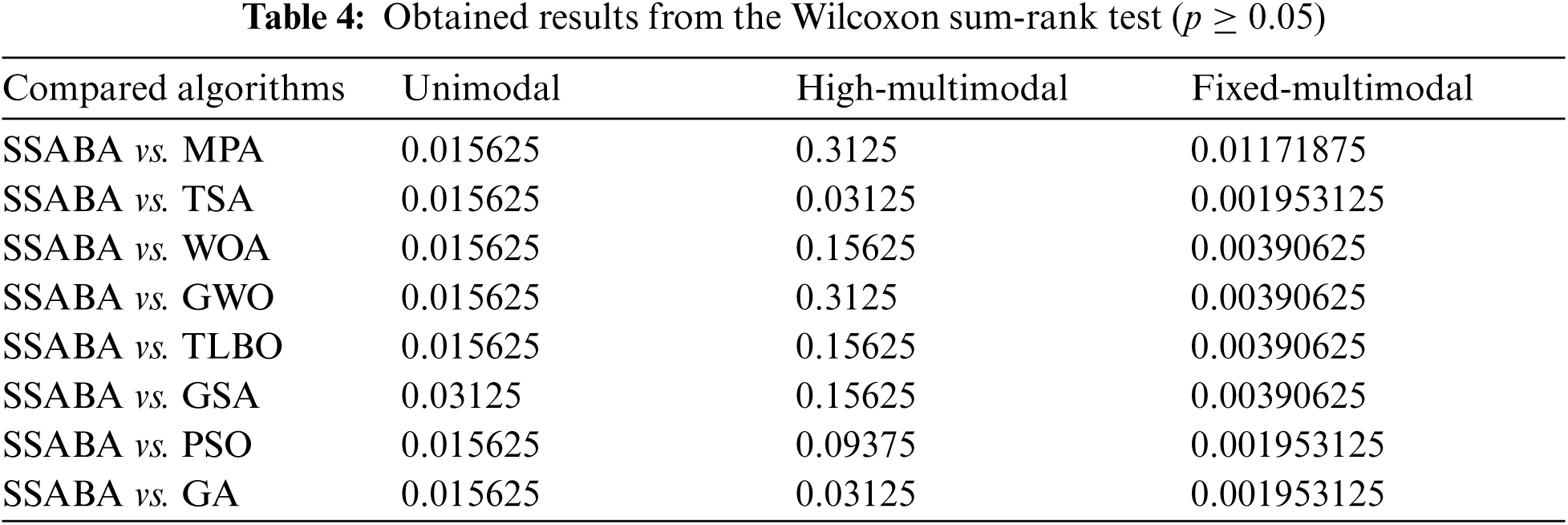4.4 Sensitivity Analysis

The proposed SSABA is a population-based algorithm that in an iteration-based process is able to provide a quasi-optimal solution for an optimization problem. Therefore, the two parameters of the maximum number of iterations and the number of population members affect the performance of the proposed algorithm. Therefore, in this subsection, the sensitivity analysis of SSABA to these two parameters is presented.

In order to provide the sensitivity analysis of SSABA to the maximum number of iterations, in independent performances, the proposed algorithm for the maximum number of iterations of 100, 500, 800, and 1000 is implemented on all twenty-three objective functions. The simulation results of this analysis are presented in Tab. 5. What can be deduced from the simulation results presented in this table is that the values of the objective functions decrease when the maximum number of iterations of the algorithm increases.

In order to provide a sensitivity analysis of the proposed algorithm to the number of population members, in independent implementations, SSABA for the number of population members 20, 30, 50, and 80 is implemented on all twenty-three objective functions. The simulation results of this analysis are presented in Tab. 6. Based on the results of the sensitivity analysis of the proposed SSABA to the number of population members, it is clear that increasing the number of population members leads to the more accurate search of the search space and thus converges the algorithm to appropriate quasi-optimal solutions which are closer to global optimal.5  Conclusions and Future Works

Numerous optimization problems designed in different sciences require optimization using appropriate methods. Optimization algorithms, which are one of the stochastic methods of problem solving, are able to provide acceptable quasi-optimal solutions to optimization problems based on random scan of the search space. In this paper, a new optimization algorithm called Search Step Adjustment Based Algorithm (SSABA) was introduced to be used in solving optimization problems. In designing the proposed SSABA, the idea of setting the search step in the problem-solving space for population members during the replication of the algorithm was applied. The step setting process is such that in the initial iterations, the search step is given the highest value in order to explore different areas of the search space. Then, as the iterations of the algorithm increase, the search steps become smaller to converge towards the optimal solution as the exploitation power of the algorithm increases. The proposed SSABA was mathematically modeled and its performance was tested on twenty-three standard functions of different types of unimodal and multimodal. The results of optimizing the unimodal functions indicated the high exploitation power of the SSABA in local search and approaching the global optimal. The results of optimizing the multimodal functions indicated the optimal capability of the SSABA in the exploration and accurate scanning of the problem search space. The performance of the proposed SSABA in providing quasi-optimal solutions was compared with eight algorithms Particle Swarm Optimization (PSO), Genetic Algorithm (GA), Teaching-Learning Based Optimization (TLBO), Gravitational Search Algorithm (GSA), Grey Wolf Optimization (GWO), Whale Optimization Algorithm (WOA), Marine Predators Algorithm (MPA), and Tunicate Swarm Algorithm (TSA). Analysis of the simulation results showed that SSABA is much more competitive compared to the eight mentioned algorithms and has a good performance in solving optimization problems of different types.

The authors offer several suggestions for future work, including the design of binary versions and multi-objective of the proposed SSABA. In addition, the use of SSABA in solving optimization problems in various sciences, nonlinear dynamic systems optimization problems, unconstrained optimization problems, and real-world problems in order to achieve appropriate solutions are from other suggestions for future researches.

Funding Statement: PT (corresponding author) and SH was supported by the Excellence project PřF UHK No. 2202/2020-2022 and Long-term development plan of UHK for year 2021, University of Hradec Králové, Czech Republic, https://www.uhk.cz/en/faculty-of-science/about-faculty/official-board/internal-regulations-and-governing-acts/governing-acts/deans-decision/2020#grant-competition-of-fos-uhk-excellence-for-2020.

Conflicts of Interest: The authors declare that they have no conflicts of interest to report regarding the present study.

References

1.  1.  M. Dehghani, Z. Montazeri, G. Dhiman, O. Malik, R. Morales-Menendez et al., “A spring searchalgorithm applied to engineering optimization problems,” Applied Sciences, vol. 10, no. 18, pp. 6173, 2020.
2.  2.  M. Dehghani, Z. Montazeri, A. Dehghani, O. P. Malik, R. Morales-Menendez et al., “Binary spring searchalgorithm for solving various optimization problems,” Applied Sciences, vol. 11, no. 3, pp. 1286, 2021.
3.  3.  S. A. Doumari, H. Givi, M. Dehghani, Z. Montazeri, V. Leiva et al., “A new two-stage algorithm for solvingoptimization problems,” Entropy, vol. 23, no. 4, pp. 491, 2021.
4.  4.  M. Dehghani, Z. Montazeri and \v{S}. Hub\'{a}lovsk\'{y}, “GMBO:Group mean-based optimizer for solving variousoptimization problems,” Mathematics, vol. 9, no. 11, pp. 1190, 2021.
5.  5.  M. Dehghani and P. Trojovský, “Teamwork optimization algorithm: A new optimization approach for function minimization/maximization,” Sensors, vol. 21, no. 13, pp. 4567, 2021.
6.  6.  F. A. Zeidabadi, S. A. Doumari, M. Dehghani and O. P. Malik, “MLBO: Mixed leader based optimizer for solving optimization problems,” International Journal of Intelligent Engineering and Systems, vol. 14, no. 4, pp. 472–479, 2021.
7.  7.  M. Dorigo, V. Maniezzo and A. Colorni, “Ant system: Optimization by a colony of cooperating agents,” IEEE Transactions on Systems, Man, and Cybernetics, Part B (Cybernetics), vol. 26, no. 1, pp. 29–41, 1996.
8.  8.  E. Rashedi, H. Nezamabadi-Pour and S. Saryazdi, “GSA: A gravitational search algorithm,” Information Sciences, vol. 179, no. 13, pp. 2232–2248, 2009.
9.  9.  M. Dehghani, Z. Montazeri, H. Givi, J. M. Guerrero and G. Dhiman, “Darts game optimizer: A new optimization technique based on darts game,” International Journal of Intelligent Engineering and Systems, vol. 13, pp. 286–294, 2020.
10. 10. M. Dehghani, Z. Montazeri, A. Dehghani, H. Samet, C. Sotelo et al., “DM: Dehghani method for modifying optimization algorithms,” Applied Sciences, vol. 10, no. 21, pp. 7683, 2020.
11. 11.  M. Dehghani, Z. Montazeri, O. P. Malik, “Energy commitment: A planning of energy carrier based on energy consumption,” Electrical Engineering & Electromechanics, no. 4, pp. 69–72, 2019. https://doi.org/10.20998/2074-272X.2019.4.10.
12. 12. M. Dehghani, M. Mardaneh, O. P. Malik, J. M. Guerrero, C. Sotelo et al., “Genetic algorithm for energy commitment in a power system supplied by multiple energy carriers,” Sustainability, vol. 12, no. 23, pp. 10053, 2020.
13. 13. M. Dehghani, M. Mardaneh, O. P. Malik, J. M. Guerrero, R. Morales-Menendez et al., “Energy commitment for a power system supplied by multiple energy carriers system using following optimization algorithm,” Applied Sciences, vol. 10, no. 17, pp. 5862, 2020.
14. 14. H. Rezk, A. Fathy, M. Aly and M. N. F. Ibrahim, “Energy management control strategy for renewable energy system based on spotted hyena optimizer,” Computers, Materials & Continua, vol. 67, no. 2, pp. 2271–2281, 2021.
15. 15. A. Ehsanifar, M. Dehghani and M. Allahbakhshi, “Calculating the leakage inductance for transformer inter-turn fault detection using finite element method,” in Proc. of Iranian Conf. on Electrical Engineering (ICEE), Tehran, Iran, pp. 1372–1377, 2017.
16. 16. M. Dehghani, Z. Montazeri and O. Malik, “Optimal sizing and placement of capacitor banks and distributed generation in distribution systems using spring search algorithm,” International Journal of Emerging Electric Power Systems, vol. 21, no. 1, pp. 20190217, 2020. https://www.degruyter.com/document/doi/10.1515/ijeeps-2019-0217/html.
17. 17. M. Dehghani, Z. Montazeri, O. P. Malik, K. Al-Haddad, J. Guerrero et al., “A new methodology called dice game optimizer for capacitor placement in distribution systems,” Electrical Engineering & Electromechanics, no. 1, pp. 61–64, 2020. https://doi.org/10.20998/2074-272X.2020.1.10.
18. 18. S. Dehbozorgi, A. Ehsanifar, Z. Montazeri, M. Dehghani and A. Seifi, “Line loss reduction and voltage profile improvement in radial distribution networks using battery energy storage system,” in Proc. of IEEE 4th Int. Conf. on Knowledge-Based Engineering and Innovation (KBEI), Tehran, Iran, pp. 215–219, 2017.
19. 19. Z. Montazeri and T. Niknam, “Optimal utilization of electrical energy from power plants based on final energy consumption using gravitational search algorithm,” Electrical Engineering & Electromechanics, no. 4, pp. 70–73, 2018. https://doi.org/10.20998/2074-272X.2018.4.12.
20. 20. M. Dehghani, M. Mardaneh, Z. Montazeri, A. Ehsanifar, M. J. Ebadi et al., “Spring search algorithm for simultaneous placement of distributed generation and capacitors,” Electrical Engineering & Electromechanics, no. 6, pp. 68–73, 2018. http://eie.khpi.edu.ua/article/view/2074-272X.2018.4.12.
21. 21. M. Premkumar, R. Sowmya, P. Jangir, K. S. Nisar and M. Aldhaifallah, “A new metaheuristic optimization algorithms for brushless direct current wheel motor design problem,” Computers, Materials & Continua, vol. 67, no. 2, pp. 2227–2242, 2021.
22. 22. M. Dehghani, Z. Montazeri, A. Ehsanifar, A. R. Seifi, M. J. Ebadi and O. M. Grechko, “Planning of energy carriers based on final energy consumption using dynamic programming and particle swarm optimization,” Electrical Engineering & Electromechanics, no. 5, pp. 62–71, 2018. https://doi.org/10.20998/2074-272X.2018.5.10.
23. 23. Z. Montazeri and T. Niknam, “Energy carriers management based on energy consumption,” in Proc. of IEEE 4th Int. Conf. on Knowledge-Based Engineering and Innovation (KBEI), Tehran, Iran, pp. 0539–0543, 2017.
24. 24. S. A. Doumari, F. A. Zeidabadi, M. Dehghani and O. P. Malik, “Mixed best members based optimizer for solving various optimization problems,” International Journal of Intelligent Engineering and Systems, vol. 14, no. 4, pp. 384–392, 2021.
25. 25. J. Kennedy and R. Eberhart, “Particle swarm optimization,” in Proc. of ICNN'95-Int. Conf. on Neural Networks, Perth, WA, Australia, pp. 1942–1948, 1995.
26. 26. S. Mirjalili, S. M. Mirjalili and A. Lewis, “Grey wolf optimizer,” Advances in Engineering Software, vol. 69, pp. 46–61, 2014.
27. 27. S. Mirjalili and A. Lewis, “The whale optimization algorithm,” Advances in Engineering Software, vol. 95, pp. 51–67, 2016.
28. 28. R. V. Rao, V. J. Savsani and D. Vakharia, “Teaching–learning-based optimization: A novel method for constrained mechanical design optimization problems,” Computer-Aided Design, vol. 43, no. 3, pp. 303–315, 2011.
29. 29. A. Faramarzi, M. Heidarinejad, S. Mirjalili and A. H. Gandomi, “Marine predators algorithm: A nature-inspired metaheuristic,” Expert Systems with Applications, vol. 152, pp. 113377, 2020.
30. 30. S. Kaur, L. K. Awasthi, A. L. Sangal and G. Dhiman, “Tunicate swarm algorithm: A new bio-inspired based metaheuristic paradigm for global optimization,” Engineering Applications of Artificial Intelligence, vol. 90, pp. 103541, 2020.
31. 31. G. Dhiman and V. Kumar, “Emperor penguin optimizer: A bio-inspired algorithm for engineering problems,” Knowledge-Based Systems, vol. 159, pp. 20–50, 2018.
32. 32. M. Dehghani, M. Mardaneh and O. Malik, “FOA: ‘following’ optimization algorithm for solving power engineering optimization problems,” Journal of Operation and Automation in Power Engineering, vol. 8, no. 1, pp. 57–64, 2020.
33. 33. M. Dehghani, M. Mardaneh, O. P. Malik and S. M. NouraeiPour, “DTO: Donkey theorem optimization,” in Proc. of Iranian Conf. on Electrical Engineering (ICEE), Yazd, Iran, pp. 1855–1859, 2019.
34. 34. M. Dehghani, Z. Montazeri, A. Dehghani, R. A. Ramirez-Mendoza, H. Samet et al., “MLO: Multi leader optimizer,” International Journal of Intelligent Engineering and Systems, vol. 13, pp. 364–373, 2020.
35. 35. H. Givi, M. Dehghani, Z. Montazeri, R. Morales-Menendez, R. A. Ramirez-Mendoza et al., “GBUO: “the good, the bad, and the ugly” optimizer,” Applied Sciences, vol. 11, no. 5, pp. 2042, 2021.
36. 36. A. Sadeghi, S. A. Doumari, M. Dehghani, Z. Montazeri, P. Trojovský et al., “A new “good and bad groups-based optimizer” for solving various optimization problems,” Applied Sciences, vol. 11, no. 10, pp. 4382, 2021.
37. 37. S. Kirkpatrick, C. D. Gelatt and M. P. Vecchi, “Optimization by simulated annealing,” Science, vol. 220, no. 4598, pp. 671–680, 1983.
38. 38. M. Dehghani, Z. Montazeri, A. Dehghani and A. Seifi, “Spring search algorithm: A new meta-heuristic optimization algorithm inspired by hooke's law,” in Proc. of IEEE 4th Int. Conf. on Knowledge-Based Engineering and Innovation (KBEI), Tehran, Iran, pp. 0210–0214, 2017.
39. 39. M. Dehghani and H. Samet, “Momentum search algorithm: A new meta-heuristic optimization algorithm inspired by momentum conservation law,” SN Applied Sciences, vol. 2, no. 10, pp. 1–15, 2020.
40. 40. A. Hatamlou, “Black hole: A new heuristic optimization approach for data clustering,” Information Sciences, vol. 222, pp. 175–184, 2013.
41. 41. A. Kaveh and M. Khayatazad, “A new meta-heuristic method: Ray optimization,” Computers & Structures, vol. 112, pp. 283–294, 2012.
42. 42. M. H. Tayarani-N and M. R. Akbarzadeh-T, “Magnetic optimization algorithms a new synthesis,” in IEEE Congress on Evolutionary Computation (IEEE World Congress on Computational Intelligence), pp. 2659–2664, Hong Kong, China, 2008.
43. 43. H. Shah-Hosseini, “Principal components analysis by the galaxy-based search algorithm: A novel metaheuristic for continuous optimisation,” International Journal of Computational Science and Engineering, vol. 6, no. 1–2, pp. 132–140, 2011.
44. 44. A. Kaveh and S. Talatahari, “A novel heuristic optimization method: Charged system search,” Acta Mechanica, vol. 213, no. 3–4, pp. 267–289, 2010.
45. 45. B. Alatas, “ACROA: Artificial chemical reaction optimization algorithm for global optimization,” Expert Systems with Applications, vol. 38, no. 10, pp. 13170–13180, 2011.
46. 46. M. Dehghani, Z. Montazeri, A. Dehghani, N. Nouri and A. Seifi, “BSSA: Binary spring search algorithm,” in Proc. of IEEE 4th Int. Conf. on Knowledge-Based Engineering and Innovation, Tehran, Iran, pp. 0220–0224, 2017.
47. 47. F. F. Moghaddam, R. F. Moghaddam and M. Cheriet, “Curved space optimization: A random search based on general relativity theory,” Arxiv Preprint Arxiv:1208.2214, 2012. https://arxiv.org/abs/1208.2214v1.
48. 48. H. Du, X. Wu and J. Zhuang, “Small-world optimization algorithm for function optimization,” in Proc. of Int. Conf. on Natural Computation, Berlin, Heidelberg, pp. 264–273, 2006.
49. 49. D. E. Goldberg and J. H. Holland, “Genetic algorithms and machine learning,” Machine Learning, vol. 3, no. 2, pp. 95–99, 1988.
50. 50. S. A. Hofmeyr and S. Forrest, “Architecture for an artificial immune system,” Evolutionary Computation, vol. 8, no. 4, pp. 443–473, 2000.
51. 51. D. Simon, “Biogeography-based optimization,” IEEE Transactions on Evolutionary Computation, vol. 12, no. 6, pp. 702–713, 2008.
52. 52. R. Storn and K. Price, “Differential evolution–a simple and efficient heuristic for global optimization over continuous spaces,” Journal of Global Optimization, vol. 11, no. 4, pp. 341–359, 1997.
53. 53. W. Banzhaf, P. Nordin, R. E. Keller and F. D. Francone, “Genetic programming: An introduction: On the automatic evolution of computer programs and its applications,” in Library of Congress Cataloging-in-Publication Data, 1st edition, vol. 27, San Francisco, CA, USA: Morgan Kaufmann Publishers, pp. 1–398, 1998.
54. 54. H. -G. Beyer and H. -P. Schwefel, “Evolution strategies–A comprehensive introduction,” Natural Computing, vol. 1, no. 1, pp. 3–52, 2002.
55. 55. M. Dehghani, Z. Montazeri, S. Saremi, A. Dehghani, O. P. Malik et al., “HOGO: Hide objects game optimization,” International Journal of Intelligent Engineering and Systems, vol. 13, no. 4, pp. 216–225, 2020.
56. 56. M. Dehghani, M. Mardaneh, J. M. Guerrero, O. Malik and V. Kumar, “Football game based optimization: An application to solve energy commitment problem,” International Journal of Intelligent Engineering and Systems, vol. 13, pp. 514–523, 2020.
57. 57. M. Dehghani, Z. Montazeri, O. P. Malik, A. Ehsanifar and A. Dehghani, “OSA: Orientation search algorithm,” International Journal of Industrial Electronics, Control and Optimization, vol. 2, no. 2, pp. 99–112, 2019.
58. 58. M. Dehghani, Z. Montazeri and O. P. Malik, “DGO: Dice game optimizer,” Gazi University Journal of Science, vol. 32, no. 3, pp. 871–882, 2019.
59. 59. S. A. Doumari, H. Givi, M. Dehghani and O. P. Malik, “Ring toss game-based optimization algorithm for solving various optimization problems,” International Journal of Intelligent Engineering and Systems, vol. 14, no. 3, pp. 545–554, 2021.
60. 60. M. Dehghani, Z. Montazeri, O. P. Malik, G. Dhiman and V. Kumar, “BOSA: Binary orientation search algorithm,” International Journal of Innovative Technology and Exploring Engineering, vol. 9, no. 1, pp. 5306–5310, 2019.
61. 61. F. Wilcoxon, “Individual comparisons by ranking methods,” Breakthroughs in Statistics, vol. 1, no. 6, pp. 196–202, 1992.

Appendix A. Objective functions

The information of the F1 to F23 objective functions used in the simulation section is specified in Tabs. A1A3.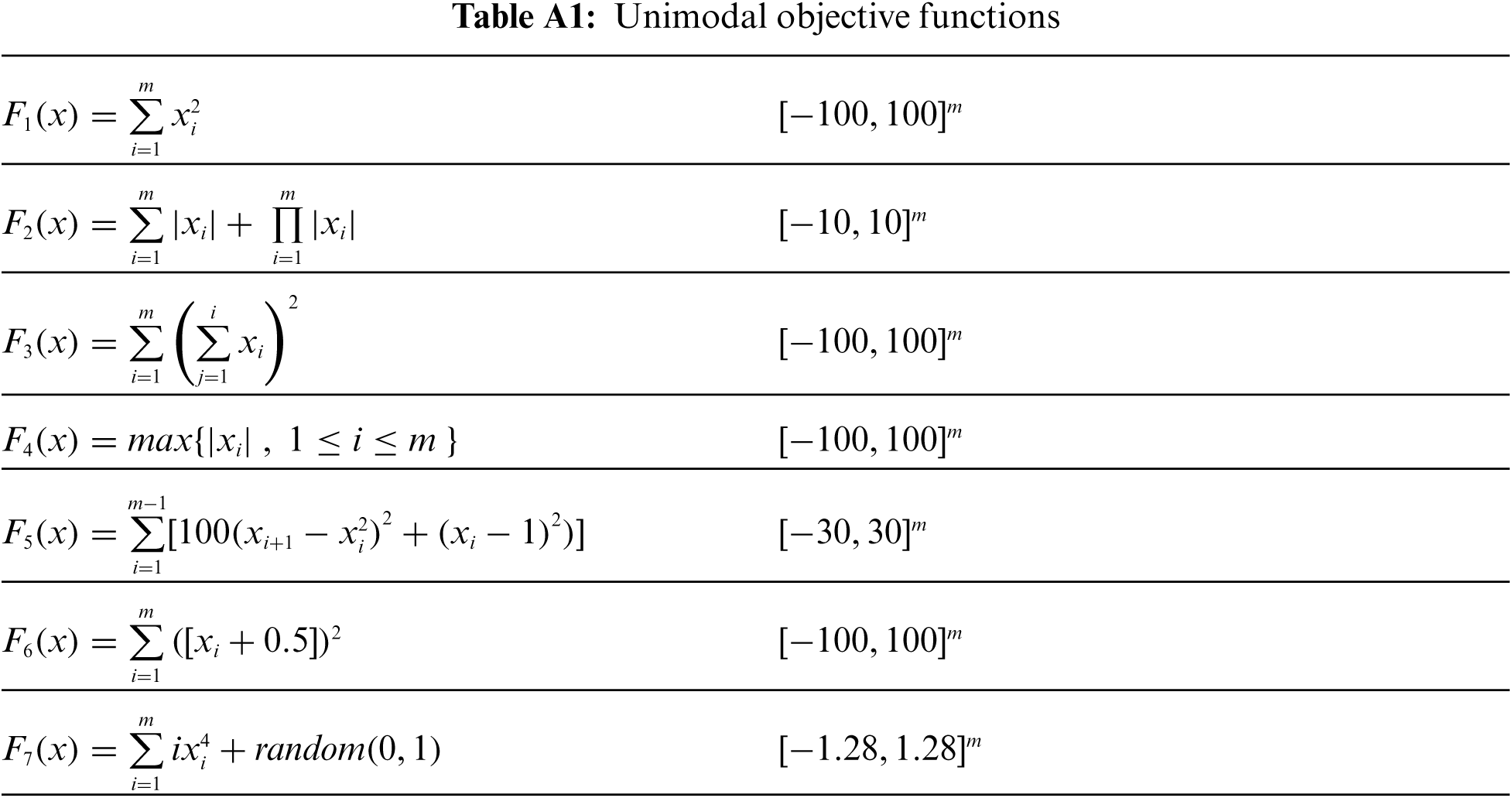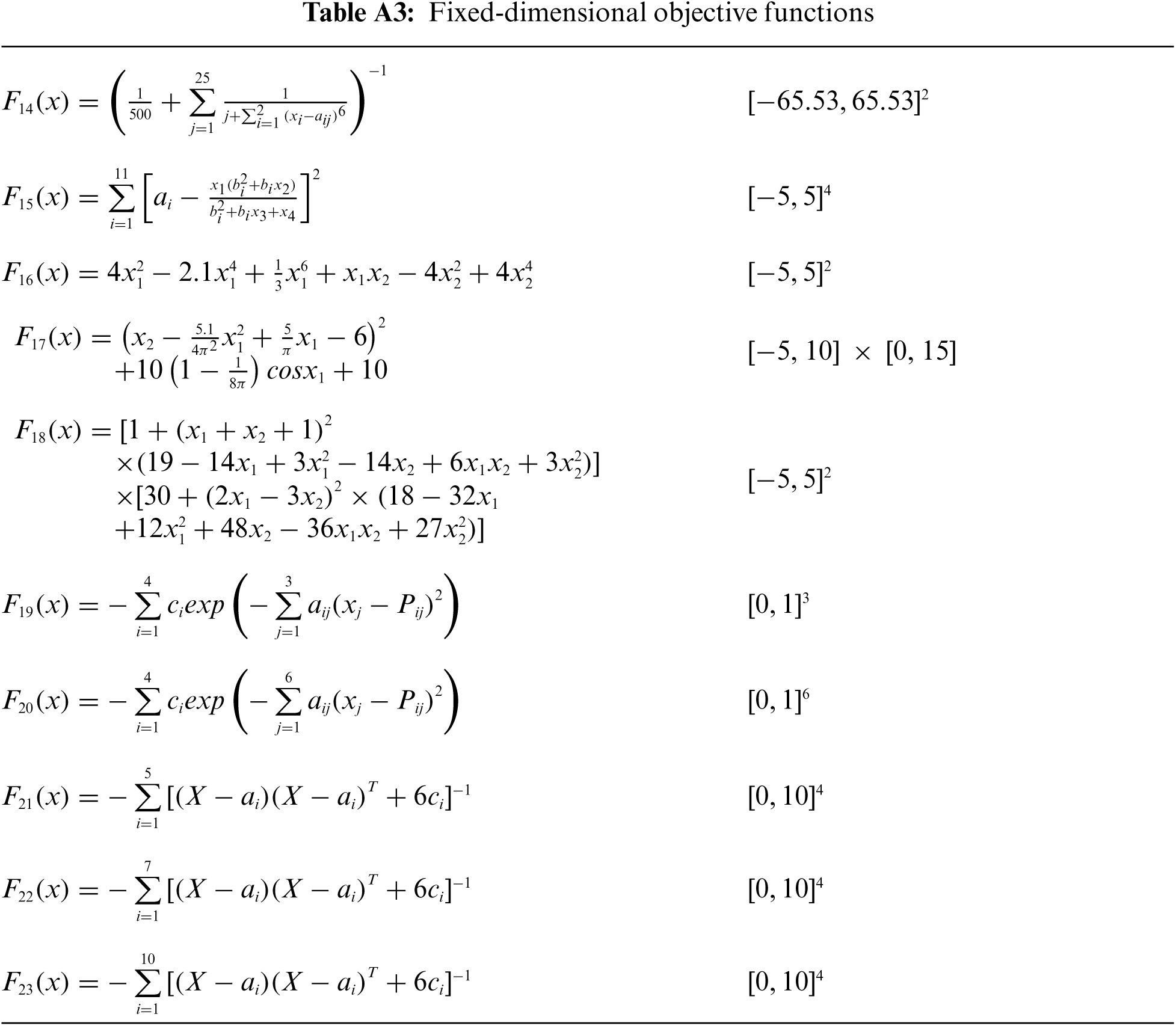This work is licensed under a Creative Commons Attribution 4.0 International License, which permits unrestricted use, distribution, and reproduction in any medium, provided the original work is properly cited.